Goseeko blog# What is Amplitude Modulation?

The amplitude of the carrier signal varies in accordance with the instantaneous amplitude of the modulating signal in Amplitude Modulation. That is the amplitude of the carrier signal containing no information varies as per the amplitude of the signal containing information, at each instant.

## Concept of Amplitude Modulation

Consider the following diagram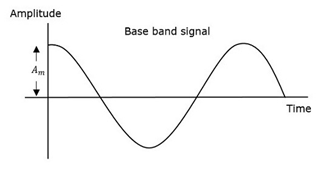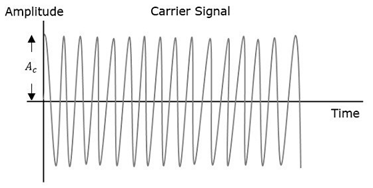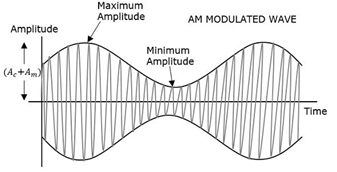The message signal shown above is in the form of a modulating wave. Then there is a carrier wave signal which has a higher frequency. This carrier wave has no information. At last shown is the modulated wave form. There is interconnection between the positive and negative ends of the carrier wave which helps to recover the exact shape of the modulating signal. This is called an Envelope. This is exactly the same as the message signal.

Therefore, we can say that Amplitude Modulation is the process of changing the amplitude of a carrier signal in accordance with the amplitude of the modulating signal. The carrier amplitude varies linearly by the modulating signal which usually consists of a range of audio frequencies. Then there is no effect on the frequency of the carrier.

## Forms

The basic forms of AM are:

• Double Sideband Large carrier modulation (DSBLC) /Double Sideband Full Carrier (DSBFC)
• Double Sideband Suppressed carrier (DSBSC) modulation.
• Single Sideband (SSB) modulation

Generation of DSB-FC (Double Side Band Full Wave Carrier)

Time-Domain Representation

Let the us consider the modulating signal as,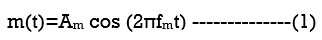Let the carrier signal be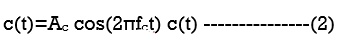Where:

Am : It is the Amplitude of the modulating signal

Ac : Amplitude of carrier signal respectively.

fm : It is the Frequency of the modulating signal

fc : The carrier signal frequency.

Then Amplitude modulated wave will be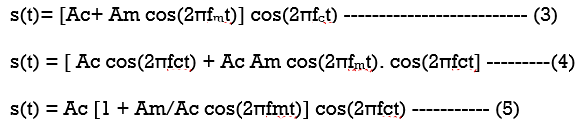Then modulation index will be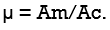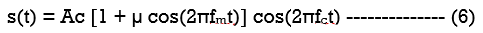Modulation index

If Amax :The maximum amplitudes of the modulated wave

Amin :The minimum amplitudes of the modulated wave.

When cos(2πfmt) is 1, We will get the maximum amplitude of the modulated wave

Amax=Ac + Am

When cos(2πfmt) is -1, We will get the minimum amplitude of the modulated wave

Amin = Ac – Am

Amax + Amin = Ac + Am + Ac –Am = 2Ac

Ac = Amax + Amin/2

Hence we can write,

Amax – Amin = Ac + Am – Ac +Am = 2Am

Am = Amax – Amin /2

Therefore  modulation index is now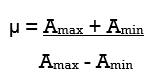## Bandwidth:

Bandwidth is the difference between the highest and lowest frequencies of the signal.

BW = fmax – fmin

s(t) = Ac [1+ μ cos(2πfmt)] cos(2πfct)

Therefore,

s(t) = Ac cos(2πfct) + Ac μ cos(2πfmt) cos(2πfct)

s(t) = Ac cos(2πfct) + Ac μ/2 cos 2π (fc + fm) t + Ac μ/2 cos 2π (fc – fm) t

The main three frequencies in amplitude modulated wave are

• Carrier frequency fc
• Upper sideband frequency fc+fm
• and lower sideband frequency fc−fm

Where we can write

fmax=fc+fm and fmin=fc−fm

BW=fc+fm−(fc−fm)

BW=fc+fm−(fc−fm)

BW= 2fm

Interested in learning about similar topics? Here are a few hand-picked blogs for you!